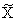General Report on Tunny

21 Page 37

---------------------------------------------------------
21   SOME PROBABILITY TECHNIQUES
---------------------------------------------------------

 (a) Symbols used in symbolic logic (b) Simple probability notations (c) Special values of p (d) Relationship of events (e) The laws of probability (f) Some theorems (including Bayes' theorem) (g) The deciban (h) Methods of applying the above axioms (i) Theorem of the weighted average of factors (j) Theorem of the chain of witnesses (k) Expected value, standard deviation, variance, distributions (l) Some special distributions (m) Some simple formulae of a non-analytic type, concerning proportional bulges (n) The general formula for sigma in Tunny work (o) The statistician's fallacy (p) The principle of maximum likelihood

It is assumed that the reader has at any rate an elementary knowledge of probability theory. Therefore the account presented here does not contain many examples but is mainly a list of definitions, notations and theorems. Rigour is deliberately avoided when it would make the account more difficult to read.

(a) Symbols used in symbolic logic.

∨ means 'or'
. means 'and', but the symbol . is often omitted, thus E.F can be written EF (E and F being propositions).
~ means 'not', but we shall write '' instead of the usual '~X'.

(b) Simple probability notations.

P(E|H) means the probability of an event E given a hypothesis H. When H is taken for granted we write P(E) simply.
The letter p represents a probability.
The letter o represents odds and is defined by the equation